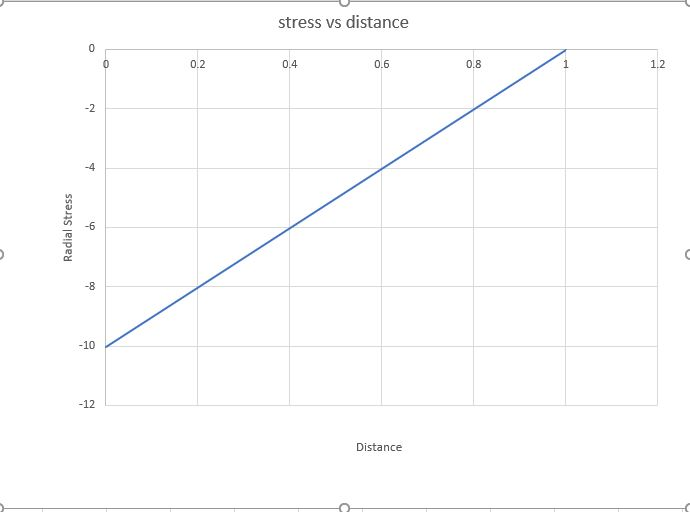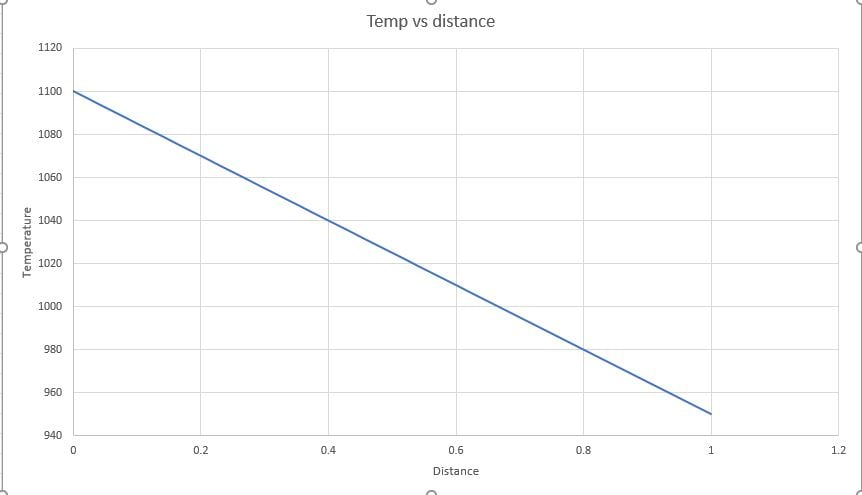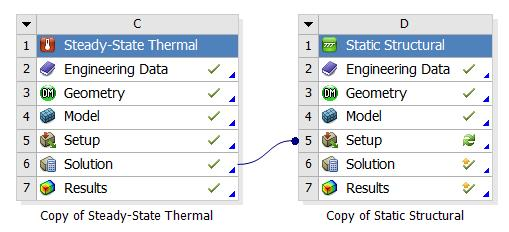# Radial stress in plane strain 2D cylinder

2»

• Sir

First i thought that i will make a model that will have 3 layers and the middle of the layer will provide some more layers that will hold the properties of the such as k2,alpha2 etc of the average of the 1st and 3rd layer.So what i did i just drew a linear line from the first and third layers and find out the properties of the middle layers from the line by plotting distance on the x axis and properties on the y axis.Because i didn't know whether ansys can make the middle layer according to the variable properties by radius or not.

1. Sir i will study according to your suggestion.But sir as i don't know how to make middle layer following the properties by the mixture rule i used in mathematica. Sir i want to make the model according to the code i gave you of the mathematica. So sir if you make a model of the picture i have attached now. I will study harder and find out new conclusions.

2.Sir you just use plane strain in your stress and deformation calculation.Further i will change it to plane stress if needed as you had learned me earlier.I hope the result will be just like the graph i had given to you earlier.Sir if you have any doubt, try to get help from the code of the mathematica. Sir try to give the video of how you make the model. I easily get the concepts then as i am still a beginner sir.

3.Sir as i am your sincere student.I will study whatever problems you face making my model and getting the similar result from that model and then i will try to solve your doubts.And you know, i have learnt all the things you have tried to deliver . I am continuing my work on the mechanical now.. Thank you very much for all your support so far.

• Because i didn't know whether ansys can make the middle layer according to the variable properties by radius or not.

Yes it can, you have to create a material with a field variable as described above.

2.Sir you just use plane strain in your stress and deformation calculation.Further i will change it to plane stress if needed as you had learned me earlier.I hope the result will be just like the graph i had given to you earlier.Sir if you have any doubt, try to get help from the code of the mathematica.

I looked at the code some more, it is written in terms of r and theta, therefore this is an axisymmetric model. What I find troubling is that there are no equations in terms of the axial direction, which might be called z (or y or x). Yet axisymmetric models have to satisfy equilibrium in all three directions. Axisymmetric equations of equilibrium are different from 2D Plane Stress and 2D Plane Strain, so forget my request to show me those equations. You should go look for a reference on the equilibrium equations for axisymmetric models and show me those equations, then compare them to the mathematica code.

• Sir

You can make 2D plane strain model. Forget this portion axisymmetric for r and theta. We just make this model just like before.I hope the result will be satisfactory.

Sir what can be done with the problem second layer? Can you help me studying out for a solution for this layer?

Regards

• You have two "black boxes" that take inputs and produce outputs. One is an ANSYS model, the other is Mathematica code that is also a model.

You can give each black box the same inputs and if they each generate the same outputs, you can say that they perform the same function inside the black box.

But what do you do when they give different outputs for the same inputs? Either there is a mistake in one model or there is no mistake, but one black box actually does not perform the same function as the other black box. For example, the ANSYS model may be built to evaluate 2D plane strain but the Mathematica model may be written to evaluate axisymmetric models.

I suggest we first find out if the two models produce the same outputs for the same inputs.  That means making K2, Alpha2, Nu2, and E2 all constants. Then we can quickly generate outputs from both ANSYS and Mathematica.  If the outputs are identical, then we can go to the next step of learning how to use Field Variables in ANSYS.  But if the outputs are different, then we have to study what is inside the black box and see what the Mathematica code is doing.

• Ok sir .

I have got you.Then SIr as you have told , i am trying to study about if the 3 layers are homogeneous then what will be the output in mathematica. And sir you try to make the model as i told in my last message.

Sir i will have no problem if the outputs are different because of the solver of ansys and matheatica. But i will not be satisfied if i make the wrong model of how the model is told on the mathematica. I am trying to compare results of the same model between the simulated on ansys and numerical solution from mathematica.

And ok Sir , i am studying of what you have told me to do.

Thanking you

• And sir you try to make the model as i told in my last message.

You make the ANSYS model and send me the archive and the Mathematica code that matches the constant material properties in the ANSYS model. I will check if the inputs match. Send the Mathematica output. I will check if the outputs match. You also compare the outputs, are they the same or different?

Sir i will have no problem if the outputs are different because of the solver of ansys and mathematica.

I will have a problem if the outputs are significantly different. There is no point implementing variable material properties for layer 2 in ANSYS if the outputs don't match.

• Ok Sir

I am trying to do as you have told.But sir how can i make the second layer on my model as i dont know how to define material properties on the second layer by the mixture rule i applied on the mathematica.

So what can i do now as you have taught me earlier how to make layers, i can make some layers in the second layer and on those layer i can vary the material properties linearly by radius. I think this wont give so many difference on the results comparing with mathematica graph. And sir i am giving you the update of mathematica results for the homogeneous cylinder layers(2D plane strain) that will help us to understand the difference of the results from the mathematica model with ansys model.

profound Regards

•  Attached is Mathematica code for a single material with the following properties:

Young's Modulus 10000 MPa
Poisson's Ratio 0.25
Thermal Conductivity 2.0 W/m/C
Thermal Expansion 1.1e-6

The geometry is for an inside radius 226 mm and outside radius 227.5 mm.

The boundary conditions are 1000 C inside and 950 C outside with a 10 MPa inside pressure.

Make an ANSYS model to these specifications and run the attached Mathematica code and see if the output agrees.

• Sir

Due to some personal problems, i am lately giving the reply. I will be in my previous flow after couple of days. I have completed the model you gave previously in workbench. I am attaching the archive file with the results of temperature and stress vs distance picture too.Profound Regards

• Thank you for the Archive. I noticed is there is only one element through the thickness. The elements used are linear PLANE183 elements, so with only one element, the stress profile through the thickness can only be a straight line. In this particular model, that is the exact solution, so you got away with one element, but in general, you almost always want a minimum of two elements through the thickness to detect curvature in the result.

I checked all the inputs to the ANSYS model, and all is correct except you selected Plane Stress and you said you wanted Plane Strain.  ANSYS won't let you change to that for the SS Thermal. To accomplish that for the Static Structural, you need to connect just the SS Thermal to the Setup cell of the Static Structural, then you can select Plane Strain.  In this particular case, the radial normal stress (x-direction) component is the same for both assumptions, but the axial normal stress (z-direction) is zero in the Plane Stress assumption but it is 466 MPa for the Plane Strain assumption. However, Mathematica is not calculating this component of stress.I assume the graphs above are from Mathematica.  If so, then they agree with the ANSYS results.

You are ready to advance to the next phase of testing the agreement. Build a 3-layer ANSYS model with 3 different constant material properties and see if Mathematica and ANSYS still give the same result.